Analytical torque computation 101

The last post covered several alternative ways to compute the torque of a motor or generator, using finite element analysis. The motivation was to increase confidence and rule out errors by re-checking the results with another method.

This post does the same for simple analytical methods. They are, after all, superior to numerical analysis in many ways. Firstly, they are staggeringly fast, and thus awesome for optimization and initial design space exploration. Secondly, they can give you a deeper understanding of the problem at hand. After all, knowing why the torque is proportional to the current is much, much more useful than realizing the same result after a numerical parameter sweep.

Anyways, onwards to the torque formulae.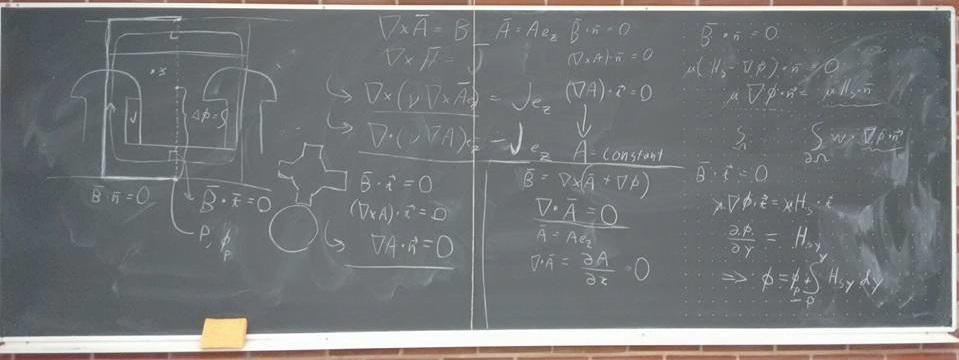Unfortunately, analytical approaches may often look as gibberish-y as this. Which is numerical stuff, by the way.

### Equivalent circuits

These are probably the first thing a motor engineer-to-be learns. Equivalent circuits, or circuit equations, for induction and synchronous machines are ubiquitous,to say the least.

And for a simple reason – they work. Have the model, and you know that the torque is proportional to an EI term of some kind. So, if you have the circuit for the machine you want, you’re pretty much set.

However, obtaining the circuit parameters in the first place can be problematic. So, the approach described next may be useful – as may the expansions and alternatives outlined in the next post.

### Simplest airgap model

This is the fundamental building block for motor design, simply. A cylindrical airgap of constant length is considered. Anything outside the gap is then reduced to surface current densities (often denoted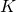) on the airgap surface – stator and rotor both.

The beauty of this approach stems from mathematics, again. By defining the surface currents suitably, it is possible to exactly represent any air-gap flux distribution. This is because (for example) the surface current acts as a boundary condition for the airgap (Neumann condition for the vector potential formulation), fixing the tangential component of the magnetic field strength. And due to the nature of Maxwell’s equations, knowing what happens on the boundary is enough info to simply neglect everything that happens elsewhere in the machine.

Then, the torque acting on the rotor (or stator) can then be obtained from the product of the radial air-gap flux density, and the surface current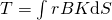.

Lorentz force, in other words.

It may not be obvious, but this approach is in fact fully equivalent to the Maxwell stress tensor method described in the last post (and in a little more detail here). After all, in cylindrical coordinates, the MST reduces to the product of the radial and circumferential flux density components. Well, the radial component is already there in the simple model, while the surface current corresponds to the tangential field strength, and hence the tangential flux density.

In practice, some simplifications are made. After all, solving the air-gap flux density exactly wouldn’t be too easy, even inside this simple cylindrical shell.

##### Simplification #1

The workaround is simple: the flux is not solved exactly. Instead, the radial flux density is assumed to equal something called the magnetomotive force divided by the gap length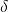and multiplied by vacuum permeability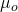. As long as the gap is short compared to its radius, no serious error is made.

The magnetomotive force – or mmf – is then the indefinite surface integral of the surface current density. This is based on the Ampere’s law, stating that the line integral of the field strength is equal to the total enclosed current. Well, here the radial flux density contributes to the line integral, while the enclosed current is of course the integral of the surface current.

In other words, between any two angular positions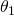and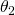we get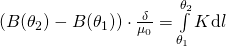Now, simply choosing to call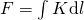we arrive at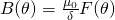In other words, mmf!

Simple, ain’t it?

##### Simplification #2

Another simplification is related to the surface currents themselves. Indeed, defining them exactly – so that the correct airgap flux could be obtained at all – is not straightforward.

Also this problem has a simple workaround – it’s not done, either! Instead, the following tricks are normally performed.

First, each winding conductor outside the airgap (naturally), is replaced by an infinitely small point-conductor on the gap surface.

Secondly, if the machine has permanent magnets, they are each represented by a pair of similar point currents, of opposite direction.

This approach also demonstrates the power of modern permanent magnets. They correspond to colossal currents. For instance, a Neodymium magnet only 5 mm high would correspond to over 5000 Ampere-turns! Something that would be quite hard to achieve with actual currents, requiring cooling and resulting in power losses.

##### Alternative illustration

I admit it – I took quite a mathematical approach in the preceding paragraphs. Thus, here’s (briefly) how the airgap model is usually taught in engineering schools.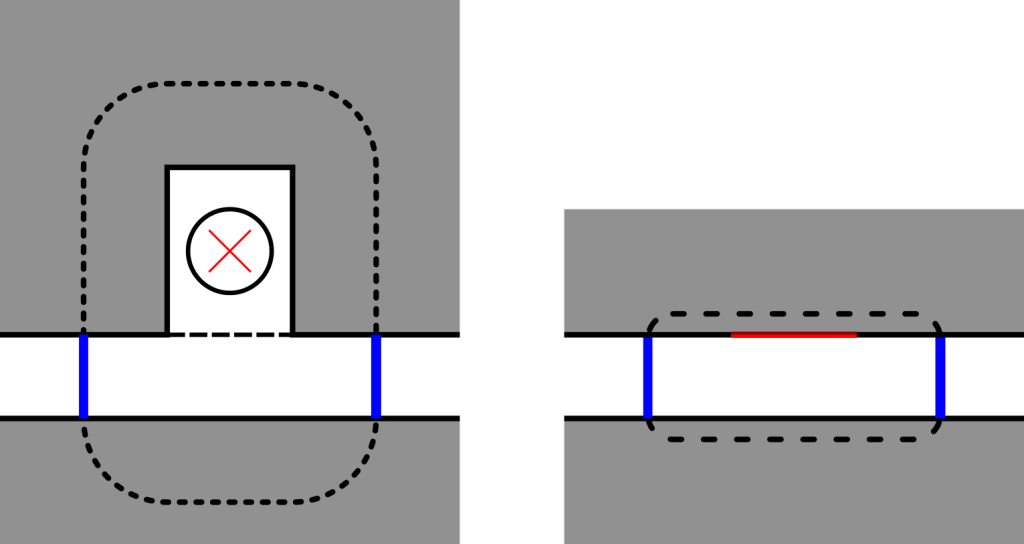Above, you can see a schematic representation of a motor sector (left subfigure). Grey is iron, white is air, and you certainly recognize the conductor symbol.

Well, the dashed line represents a closed line integral, along which the field strength H is integrated. As iron has a high permeability, only the parts in the airgap, annotated with blue, actually contribute anything to the integral. So, their sum has to equal the current flowing in the conductor.

Then, the right subfigure then represents the typical engineering-school model. The slot has been replaced with iron, and the conductor has been squeezed into an infinitely thin current sheet marked with red.

Now, here’s the important point: both figures are fully equivalent, as far as the line integral is considered. Both will yield the same field strength in the airgap, on the blue lines.

Furthermore, in the right subfigure, the iron parts contribute nothing to the integral, due to their high permeability. So, they could be replaced with nothing. This would correspond to the earlier explanation, with the surface currents acting as boundary conditions, with no attention paid to whatever happens outside the airgap.

### Options with the simple airgap model

Alright, now we know how to set up the surface currents, compute the flux density, and then use both to compute the torque. So, we’re set, right?

Not so fast. There are several simplifications to be made.

After all, we are mostly interested in the average torque here. (Besides, this simplest approach is not very good for computing torque ripple – more of this in the next post). Additionally, our machine probably has roughly sinusoidal phase currents.

For that reason, the airgap flux and surface currents are typically also written as a Fourier series, of both timeand angle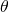.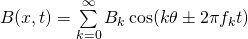Then, only the components with the correct number of poles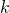and the correct frequency will contribute to the torque. For instance, in four-pole machine supplied with 50 Hz sinusoidal currents, only those flux waves with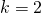and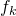= 50 Hz would create useful torque.

But, there’s a lot of leeway here. For instance, it’s possible to assume the airgap flux density to be rectangular, but only consider sinusoidal surface currents. Or vice versa. Or keep the original point currents. Or any other combination you can think of.

The point here is, all approaches should yield the same average torque. If they don’t, you are using at least one of them incorrectly. In that case, hope it’s the one you used for verification, rather than the design itself.

Part 2 of the analytical methods coming soon.

Check out EMDtool - Electric Motor Design toolbox for Matlab.

Need help with electric motor design or design software? Let's get in touch - satisfaction guaranteed!Options for (analytical) torque computation – Part 1